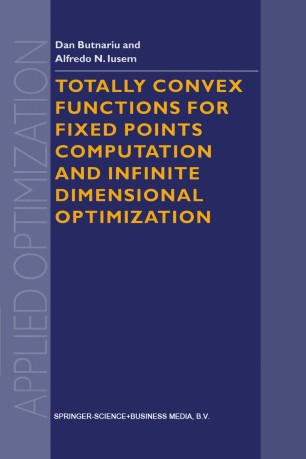# Totally Convex Functions for Fixed Points Computation and Infinite Dimensional Optimization

• Dan Butnariu
• Alfredo N. IusemBook

Part of the Applied Optimization book series (APOP, volume 40)

1. Front Matter
Pages i-xvi
2. Dan Butnariu, Alfredo N. Iusem
Pages 1-64
3. Dan Butnariu, Alfredo N. Iusem
Pages 65-128
4. Dan Butnariu, Alfredo N. Iusem
Pages 129-188
5. Back Matter
Pages 189-205

### Introduction

The aim of this work is to present in a unified approach a series of results concerning totally convex functions on Banach spaces and their applications to building iterative algorithms for computing common fixed points of mea­ surable families of operators and optimization methods in infinite dimen­ sional settings. The notion of totally convex function was first studied by Butnariu, Censor and Reich  in the context of the space lRR because of its usefulness for establishing convergence of a Bregman projection method for finding common points of infinite families of closed convex sets. In this finite dimensional environment total convexity hardly differs from strict convexity. In fact, a function with closed domain in a finite dimensional Banach space is totally convex if and only if it is strictly convex. The relevancy of total convexity as a strengthened form of strict convexity becomes apparent when the Banach space on which the function is defined is infinite dimensional. In this case, total convexity is a property stronger than strict convexity but weaker than locally uniform convexity (see Section 1.3 below). The study of totally convex functions in infinite dimensional Banach spaces was started in  where it was shown that they are useful tools for extrapolating properties commonly known to belong to operators satisfying demanding contractivity requirements to classes of operators which are not even mildly nonexpansive.

### Keywords

Banach Space Convexity Dimension Integral equation Optimal control algorithms control functional analysis linear optimization optimization quality

#### Authors and affiliations

• Dan Butnariu
• 1
• Alfredo N. Iusem
• 2
1. 1.Department of MathematicsUniversity of HaifaIsrael
2. 2.The Institute of Pure and Applied Mathematics (IMPA)Rio de JaneiroBrazil

### Bibliographic information

• DOI https://doi.org/10.1007/978-94-011-4066-9